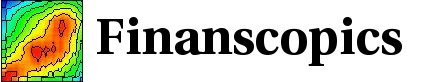Base information

### Returns and volatilities

#### Returns

For the empirical data, the empirical prices p(t) are first filtered for outliers.

The return measured at a time scale δtr is given by the logarithmic price difference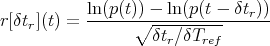(1)

with δtr the time interval used to measure the return, and ln(p) the logarithm of the prices. This is a ”historical” return definition.

The denominator is used to annualize the return using a Gaussian random walk scaling, and we take δTref = 1 year. In this way, the returns can be compared across different time horizons δtr. For example E[r[δtr]2] 10% regardless of δt r for most of the empirical time series. Similarly, the probability density p(r[δtr]) can be directly compared. When studying scaling properties, this return definition already eliminates the random walk scaling. Hence, for an iid random walk, the annualization leads to the scaling relation E[r[δtr]k] constant, whereas a simple price difference leads to E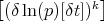δtk∕2.

#### Volatilities

The historical volatility σh at time t measures the price fluctuation at the time horizon δtr, in a window of length δtσ in the past of t: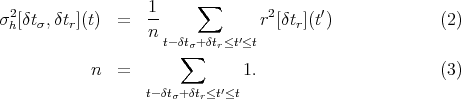Because the returns are already annualized, the volatility is also annualized.

A weight given by the tick density is implicit in this summation (in the continuum, the weight corresponds to the integration measure on the time axis). Usually, the price time series is sample regularly in some time scale, giving a uniform weight in the chosen time scale. The canonical example is the business time scale where the data are sampled regularly according to the physical time, except on the week-end and holidays.

The volatility definition involves two parameters δtr and δtσ. The most important parameter is δtσ, which fixes the time interval over which the volatility is estimated. In practice, for a given δtσ, the volatility has a weak dependency of δtr. Most of the figures are computed with a fixed ratio δtσ∕δtr = 24, namely by aggregating simultaneously the return and volatility. This choice corresponds roughly to our intuition that short term intra-day traders use tick-by-tick data, while long term traders use daily data to make decisions. In order to explore the δtr dependency, in the pages ”fine grained vs coarse grained volatility”, the parameters δtσ is kept fixed while δtr is changed.

#### Volatility increments

The historical volatility increment is defined by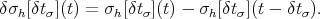(4)

It is measuring the volatility changes occurring at a time scale δtσ. Indeed, for a stochastic variable evolving on a typical time scale δtσ, this is the meaningful definition to evaluate the “changes” (and not to use a continuum derivative). Another definition of the historical volatility increment is to compute relative changes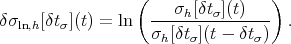(5)

The correlations computed with both definitions 4 and 5 are almost identical. The correlation figures shown in this site correspond to the definition 5 for the logarithmic increment.

For a given time t, the return, volatility and volatility increment defined above are historical quantities, as they use information only in the past of t. The corresponding ”realized” quantity is obtained by a simple time translation, so that only prices in the future of t are used.

We will use also the ”centered” volatility increment defined by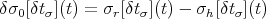(6)

and similarly for the logarithmic centered volatility increment. These centered volatility increments measure the change of volatility occurring around t, and uses the information in the past and the future of t.